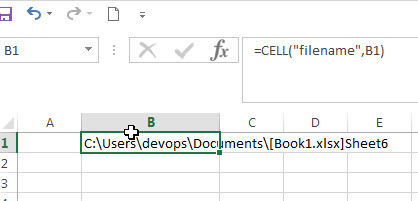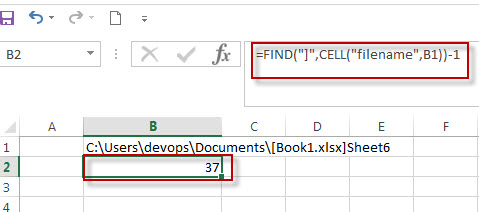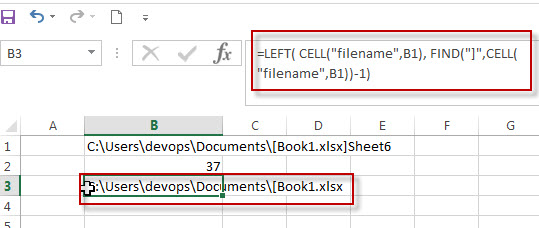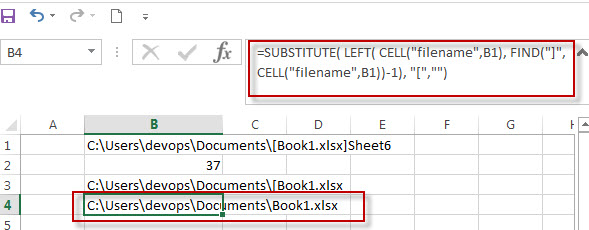# Get Path and Workbook name only

Generally, the Cell function returns the full file name contain workbook and worksheet name and it is absolute path.

If you want to get the workbook name and its path only without worksheet name, you can use a combination of the SUBSTITUTE function, the LEFT function, the CELL function and the FIND function.

`=SUBSTITUTE( LEFT( CELL("filename",B1), FIND("]",CELL("filename",B1))-1), "[","")`

The CELL function will get the full information of the current worksheet contain the full file name and its path.

`=CELL("filename",B1)`As you just want to extract the string that contain only the path and workbook name, so using the FIND function to get the position of the right bracket symbol. Then you can get the length of the path and workbook name only using the below Find formula:

`=FIND("]",CELL("filename",B1))-1`The LEFT function will get the leftmost the text characters based on the length of the string returned by the above FIND formula.

`=LEFT( CELL("filename",B1), FIND("]",CELL("filename",B1))-1)`Last, you still need to use SUBSTITUTE to replace the left bracket symbol within the result returned by the first CELL function.

`=SUBSTITUTE( LEFT( CELL("filename",B1), FIND("]",CELL("filename",B1))-1), "[","")`### Related Formulas

• Get the Current Worksheet Name only
If you want to get the current worksheet name only in excel, you can use a combination of the MID function, the CELL function and FIND function.…
• Get full File Name (workbook and worksheet) and Path
In excel, you can get the current workbook name and it is absolute path using the CELL function.  Just refer to the following formula:=CELL(“filename”,B1).…
• Get the Current Workbook Name
If you want to get the name of the current workbook only, you can use a combination of the MID function, the CELL function and the FIND Function…

### Related Functions

• Excel Find function
The Excel FIND function returns the position of the first text string (substring) from the first character of the second text string.The FIND function is a build-in function in Microsoft Excel and it is categorized as a Text Function.The syntax of the FIND function is as below:= FIND  (find_text, within_text,[start_num])…
• Excel LEFT function
The Excel LEFT function returns a substring (a specified number of the characters) from a text string, starting from the leftmost character.The LEFT function is a build-in function in Microsoft Excel and it is categorized as a Text Function.The syntax of the LEFT function is as below:= LEFT(text,[num_chars])…
• Excel CELL function
The Excel CELL function returns information about the formatting, location, size, or contents of a cell.The syntax of the CELL function is as below:= CELL (info_type,[reference])…
• Excel Substitute function
The Excel SUBSTITUTE function replaces a new text string for an old text string in a text string.The syntax of the SUBSTITUTE function is as below:= SUBSTITUTE  (text, old_text, new_text,[instance_num]) ….